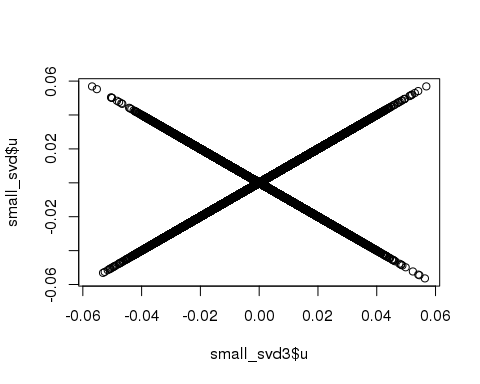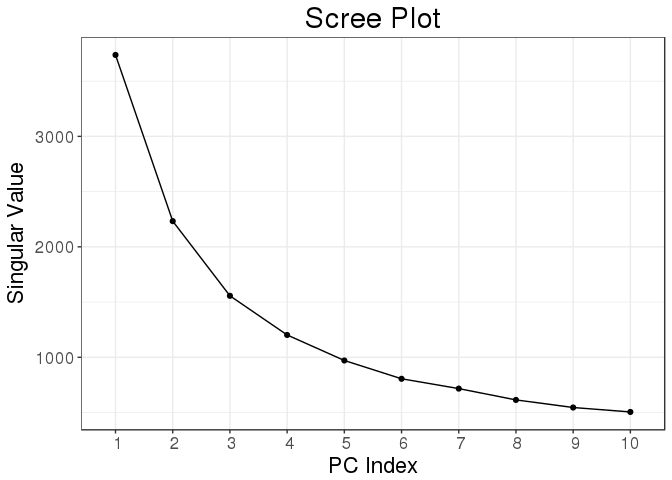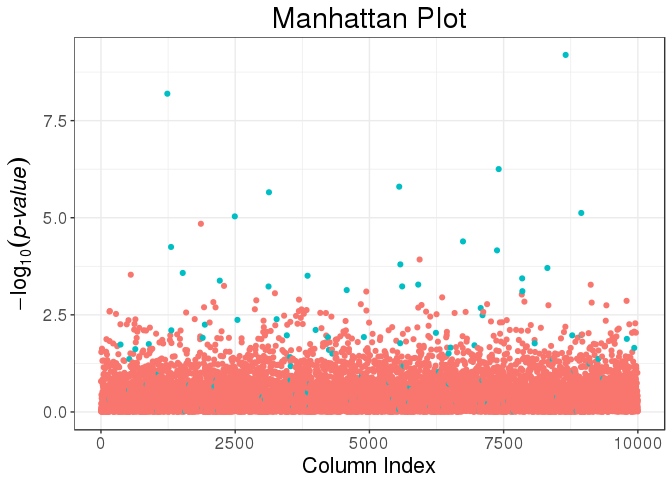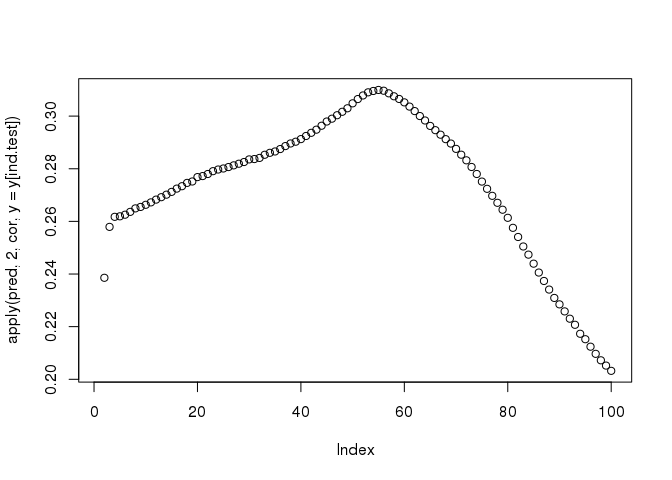In this post, I will talk about my package bigstatsr, which I’ve just presented in a lightning talk of 5 minutes at useR!2017. You can listen to me in action there. I should have chosen a longer talk to explain more about this package, maybe next time. I will use this post to give you a more detailed version of the talk I gave in Brussels.

## Motivation behind bigstatsr

I’m a PhD student in predictive human genetics. I’m basically trying to predict someone’s risk of disease based on their DNA mutations. These DNA mutations are in the form of large matrices so that I’m currently working with a matrix of 15K rows and 300K columns. This matrix would take approximately 32GB of RAM if stored as a standard R matrix.

When I began studying this dataset, I had only 8GB of RAM on my computer. I now have 64GB of RAM but it would take only copying this matrix once to make my computer begin swapping and therefore slowing down. I found a convenient solution by using the object `big.matrix` provided by the R package bigmemory (Kane, Emerson, and Weston 2013). With this solution, you can access a matrix that is stored on disk almost as if it were a standard R matrix in memory.Yet, for these special matrices, some useful statistical functions were missing or not fast enough. So, I implemented these. It was a good experience about programming optimized and parallelized algorithms. I’m aware that there are other packages that come with bigmemory, such as biganalytics and bigalgebra, that already implement some of the features I will talk about in this post. I will discuss why I don’t use these packages. However, I use the work of other packages such as biglasso and RSpectra.

## Introduction to bigmemory

```# loading package bigstatsr (and bigmemory)
library(bigstatsr)
# initializing some matrix on disk: wrapper to bigmemory::big.matrix()
mat <- FBM(backingroot = "matrix-on-disk", descriptor = FALSE)(5e3, 10e3)
## Creating directory "backingfiles" which didn't exist..
dim(mat)
##   5000 10000
mat[1:5, 1:5]
##      [,1] [,2] [,3] [,4] [,5]
## [1,]    0    0    0    0    0
## [2,]    0    0    0    0    0
## [3,]    0    0    0    0    0
## [4,]    0    0    0    0    0
## [5,]    0    0    0    0    0
mat[1, 1] <- 2
mat[1:5, 1:5]
##      [,1] [,2] [,3] [,4] [,5]
## [1,]    2    0    0    0    0
## [2,]    0    0    0    0    0
## [3,]    0    0    0    0    0
## [4,]    0    0    0    0    0
## [5,]    0    0    0    0    0
mat[2:4] <- 3
mat[1:5, 1:5]
##      [,1] [,2] [,3] [,4] [,5]
## [1,]    2    0    0    0    0
## [2,]    3    0    0    0    0
## [3,]    3    0    0    0    0
## [4,]    3    0    0    0    0
## [5,]    0    0    0    0    0
mat[, 2:3] <- rnorm(2 * nrow(mat))
mat[1:5, 1:5]
##      [,1]       [,2]       [,3] [,4] [,5]
## [1,]    2 -0.1611891  3.1218981    0    0
## [2,]    3 -0.6026680 -1.3935111    0    0
## [3,]    3  0.4743703 -0.6566549    0    0
## [4,]    3  0.1514252  0.8247594    0    0
## [5,]    0 -1.5167186  0.7329260    0    0```

What we can see is that big matrices (`big.matrix` objects) can be accessed (read/write) almost as if they were standard R matrices, but you have to be cautious. For example, doing `mat[1, ]` isn’t recommended. Indeed, big matrices, as standard R matrices, are stored by column so that it is in fact a big vector with columns stored one after the other, contiguously. So, accessing the first row would access elements that are not stored contiguously in memory, which is slow. One should always access columns rather than rows.

## Apply an R function to a big matrix

An easy strategy to apply an R function to a big matrix would be the split-apply-combine strategy (Wickham 2011). For example, you could access only a block of columns at a time, apply a (vectorized) function to this block, and then combine the results of all blocks. This is implemented in function `big_apply()`.```# Compute the sums of the first 1000 columns
colsums_1 <- colSums(mat[, 1:1000])
# Compute the sums of the second block of 1000 columns
colsums_2 <- colSums(mat[, 1001:2000])
# Combine the results
colsums_1_2 <- c(colsums_1, colsums_2)
# Do this automatically with big_apply()
colsums_all <- big_apply(mat, a.FUN = function(X, ind) colSums(X[, ind]),
a.combine = 'c')```

When the split-apply-combine strategy can be used for a given function, you could use `big_apply()` to get the results, while accessing only small blocks of columns (or rows) at a time. Package biganalytics, by the creators of bigmemory, provides a way to apply an R function to margins of a `big.matrix`. Yet, for example, if the `big.matrix` has a lot of columns, it would be much slower to loop through all columns rather that applying a vectorized function to blocks of columns. You can find more examples of applications for `big_apply()` there.

```colsums_all2 <- biganalytics::apply(mat, 2, sum)
all.equal(colsums_all2, colsums_all)
##  TRUE```

## Use Rcpp with a big matrix

Using Rcpp with a `big.matrix` is super easy. Let’s use the previous example, i.e. the computation of the colsums of a `big.matrix`. We will do it in 3 different ways.

### 1. Using the bigmemory way

```// [[Rcpp::depends(bigmemory, BH)]]
#include <bigmemory/MatrixAccessor.hpp>
#include <Rcpp.h>

using namespace Rcpp;

// [[Rcpp::export]]
NumericVector bigcolsums(const S4& BM) {

MatrixAccessor<double> macc(*xpMat);

int n = macc.nrow();
int m = macc.ncol();

NumericVector res(m); // vector of m zeros
int i, j;

for (j = 0; j < m; j++)
for (i = 0; i < n; i++)
res[j] += macc[j][i];

return res;
}
colsums_all3 <- bigcolsums(mat)
all.equal(colsums_all3, colsums_all)
##  TRUE```

### 2. Using the bigstatsr way

```// [[Rcpp::depends(bigstatsr, bigmemory, BH)]]
#include <bigstatsr/SubMatAcc.h>

// [[Rcpp::export]]
NumericVector bigcolsums2(const S4& BM,
const IntegerVector& rowInd,
const IntegerVector& colInd) {

// C++ indices begin at 0
IntegerVector rows = rowInd - 1;
IntegerVector cols = colInd - 1;
// An accessor of only part of the big.matrix
SubMatAcc<double> macc(*xpMat, rows, cols);

int n = macc.nrow();
int m = macc.ncol();

NumericVector res(m); // vector of m zeros
int i, j;

for (j = 0; j < m; j++)
for (i = 0; i < n; i++)
res[j] += macc(i, j);

return res;
}
colsums_all4 <- bigcolsums2(mat, rows_along(mat), cols_along(mat))
all.equal(colsums_all4, colsums_all)
##  TRUE```

In bigstatsr, most of the functions have parameters for subsetting rows and columns because it is often useful. One of the main reasons why I don’t use package bigalgebra is its lack of subsetting options.

### 3. Use an already implemented function

```str(colsums_all5 <- big_colstats(mat))
## 'data.frame':    10000 obs. of  2 variables:
##  \$ sum: num  11 139.6 73.2 0 0 ...
##  \$ var: num  0.0062 1.0117 1.0164 0 0 ...
all.equal(colsums_all5\$sum, colsums_all)
##  TRUE```

## Principal Component Analysis

Let’s begin by filling the matrix with random numbers in a tricky way.

```U <- sweep(matrix(rnorm(nrow(mat) * 10), ncol = 10), 2, 1:10, "/")
V <- matrix(rnorm(ncol(mat) * 10), ncol = 10)
big_apply(mat, a.FUN = function(X, ind) {
X[, ind] <- tcrossprod(U, V[ind, ]) + rnorm(nrow(X) * length(ind))
NULL
}, a.combine = 'c')
## NULL```

Let’s say we want the first 10 PCs of the (scaled) matrix.

```system.time(
small_svd <- svd(scale(mat[, 1:2000]), nu = 10, nv = 10)
)
##    user  system elapsed
##   8.348   0.063   8.429
system.time(
small_svd2 <- big_SVD(mat, big_scale(), ind.col = 1:2000)
)
## (2)
##    user  system elapsed
##   1.900   0.083   1.989
plot(small_svd2\$u, small_svd\$u)``````system.time(
small_svd3 <- big_randomSVD(mat, big_scale(), ind.col = 1:2000)
)
##    user  system elapsed
##   0.353   0.002   0.355
plot(small_svd3\$u, small_svd\$u)``````system.time(
svd_all <- big_randomSVD(mat, big_scale())
)
##    user  system elapsed
##   2.267   0.001   2.268
plot(svd_all)```Function `big_randomSVD()` uses Rcpp and package Rpsectra to implement a fast Singular Value Decomposition for a `big.matrix` that is linear in all dimensions (standard PCA algorithm is quadratic in the smallest dimension) which makes it very fast even for large datasets (that have both dimensions that are large).

## Some linear models

```M <- 100 # number of causal variables
set <- sample(ncol(mat), M)
y <- mat[, set] %*% rnorm(M)
y <- y + rnorm(length(y), sd = 2 * sd(y))

ind.train <- sort(sample(nrow(mat), size = 0.8 * nrow(mat)))
ind.test <- setdiff(rows_along(mat), ind.train)

mult_test <- big_univLinReg(mat, y[ind.train], ind.train = ind.train,
covar.train = svd_all\$u[ind.train, ])
library(ggplot2)
plot(mult_test) +
aes(color = cols_along(mat) %in% set) +
labs(color = "Causal?")``````train <- big_spLinReg(mat, y[ind.train], ind.train = ind.train,
covar.train = svd_all\$u[ind.train, ],
alpha = 0.5)
pred <- predict(train, X. = mat, ind.row = ind.test, covar.row = svd_all\$u[ind.test, ])
plot(apply(pred, 2, cor, y = y[ind.test]))```The functions `big_spLinReg()`, `big_spLogReg()` and `big_spSVM()` all use lasso (L1) or elastic-net (L1 & L2) regularizations in order to limit the number of predictors and to accelerate computations thanks to strong rules (R. Tibshirani et al. 2012). The implementation of these functions are based on modifications from packages sparseSVM and biglasso (Zeng and Breheny 2017). Yet, these models give predictions for a range of 100 different regularization parameters whereas we are only interested in one prediction.

So, that’s why I came up with the idea of Cross-Model Selection and Averaging (CMSA), which principle is:

1. This function separates the training set in K folds (e.g. 10).
2. In turn,
• each fold is considered as an inner validation set and the others (K - 1) folds form an inner training set,
• the model is trained on the inner training set and the corresponding predictions (scores) for the inner validation set are computed,
• the vector of scores which maximizes `feval` is determined,
• the vector of coefficients corresponding to the previous vector of scores is chosen.
3. The K resulting vectors of coefficients are then combined into one vector.
```train2 <- big_CMSA(big_spLinReg, feval = function(pred, target) cor(pred, target),
X. = mat, y.train = y[ind.train], ind.train = ind.train,
covar.train = svd_all\$u[ind.train, ],
alpha = 0.5, ncores = parallel::detectCores() / 2)
mean(train2 != 0) # percentage of predictors
##  0.1806194
pred2 <- predict(train2, X. = mat, ind.row = ind.test, covar.row = svd_all\$u[ind.test, ])
cor(pred2, y[ind.test])
##  0.311157```

## Some matrix computations

For example, let’s compute the correlation of the first 2000 columns.

```system.time(
corr <- cor(mat[, 1:2000])
)
##    user  system elapsed
##  10.822   0.007  10.824
system.time(
corr2 <- big_cor(mat, ind.col = 1:2000)
)
##    user  system elapsed
##   0.729   0.005   0.737
all.equal(corr2, corr)
##  TRUE```

## Advantages of using big.matrix objects

• you can apply algorithms on 100GB of data,
• you can easily parallelize your algorithms because the data on disk is shared,
• you write more efficient algorithms,
• you can use different types of data, for example, in my field, I’m storing my data with only 1 byte per element (rather than 8 bytes for a standard R matrix).

In a next post, I’ll try to talk about good practices on how to use parallelism in R.